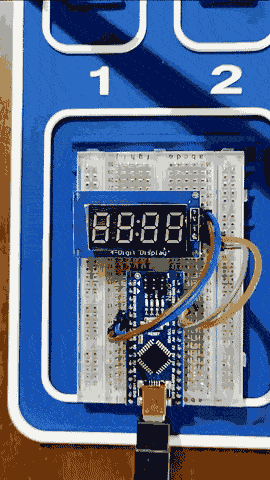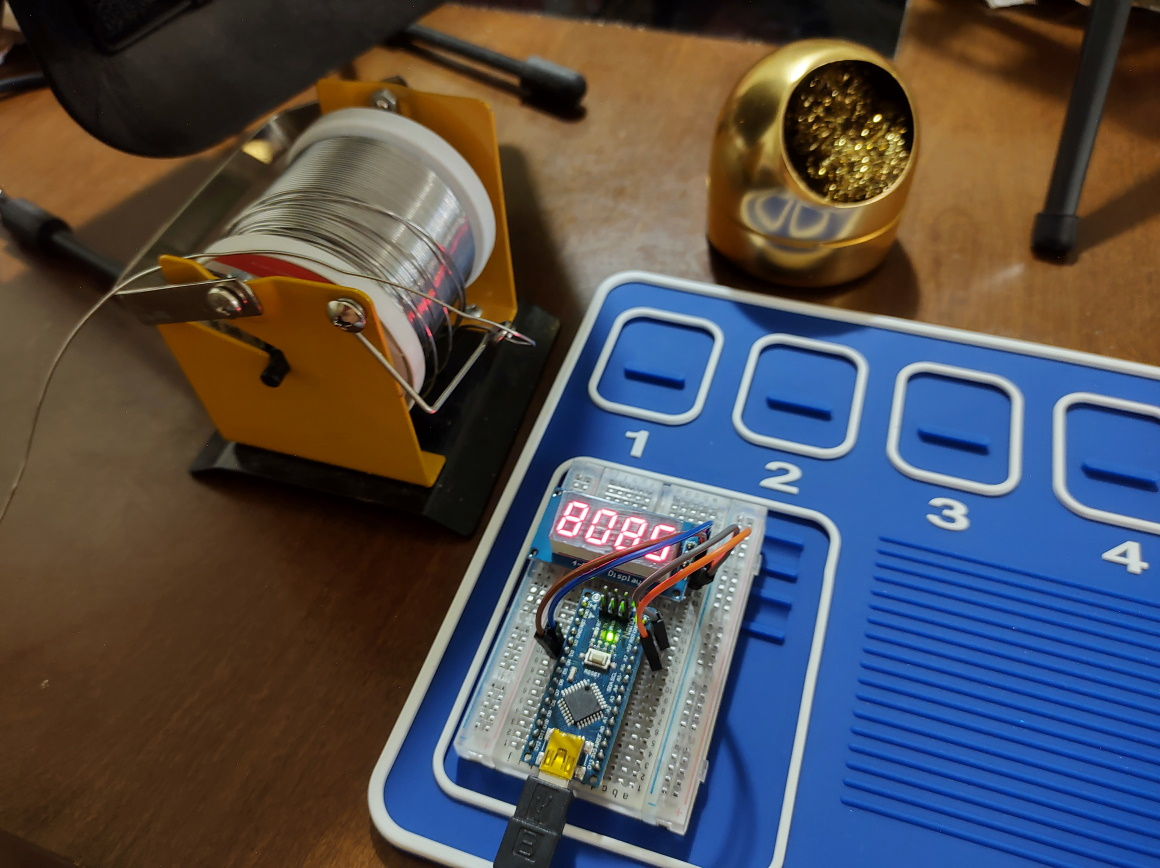# ArduinoPiMachine

Arduino Pi Machine – Calculate Pi to nth DecimalAn Arduino Nano assembly equipped with a 4 digits 7-segments led display which will, while powered, keep calculating Pi digits and scrolling through the display, like a window scrolling left to right through the infinitude of Pi digits.

For that task, I’m using Xavier Gourdon algorithm described in his “Computation of the n-th decimal digit of π with low memory” paper and the Xavier Gourdon and Pascal Sebah paper called “N-th digit computation” that comes with a nice C++ implementation example that I ported to Arduino frameworkWhen compared to a version running on PC, this code on Arduino Nano has an approximation error on the last 2-3 digits each interaction, rendering only the first 3-5 digits useable (the algorithm produces 6 digits, counting left to right from the starting position). Believe this is due to double type on ATMEGA occupying only 4 bytes. Further refinement of the code is needed for ATMEGA.

References:

http://numbers.computation.free.fr/Constants/Algorithms/nthdigit.html

http://numbers.computation.free.fr/Constants/Algorithms/nthdecimaldigit.pdf

View Github